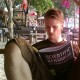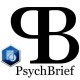# Notes on Paul Meehl’s “Philosophical Psychology Session” #02

These are the notes I made whilst watching the video recording of Paul Meehl’s philosophy of science lectures. This is the second episode (a list of all the videos can he found here). Please note that these posts are not designed to replace or be used instead of the actual videos (I highly recommend you watch them). They are to be read alongside to help you understand what was said. I also do not include everything that he said (just the main/most complex points).

##### Popper, Bayes theorem, & Lakatos

Popper did not accept the verifiable criterion of meaning. Popper never said falsifiability was a criterion of meaning.

No experimental/quantitative for Freud (there is empirical data). Popper rejected induction completely.

Unscientific theories don’t give you examples of things that will show it’s wrong, just what will confirm it.

If P > Q (conditional) = -P (P is false) v Q (Q is true) = not true that P (P is true) v -Q (Q is false). P is sufficient for Q and Q is necessary for P.

If there is a semantic connection between propositions, use stronger notation I-

Implicative syllogism: P -> Q, P therefore Q. Valid figure. Used when a theory is predicting an event. Modus ponens.

P -> Q, ~P therefore ~Q. Invalid. If Nixon is honest I’ll eat my hat. Nixon isn’t honest, can’t conclude I won’t eat my hat.

Q -> P, P therefore Q. Invalid. All inductive reasoning is formally invalid (if the theory is true then the facts will be so. The facts are so, therefore the theory is true). Hence why all empirical reasoning is probable. Hence why it can never be proved in sense of Euclid. Used when a piece of evidence is trying to support a law.

P -> Q, ~Q therefore ~P. Valid. If Newton is right, then the star will be here. The star is not here, therefore Newton is wrong. Used to refute a scientific law or theory.  Modus Tollens (destructive mood). 4th figure of implicative syllogism.

Facts control the theory collectively over the long-haul (rather than just being dismissed after one piece of counter evidence). If the theory is robust enough/substantiated enough, allowed to roll with a piece of counter-evidence. There’s no specified point where this theoretical tenacity becomes unscientific.

Empirical science cannot be like formal set theory/ mathematics as it deals with probabilities.

Demarcation of scientific theory from non-science.

We don’t just state if a theory has been “slain” or not. There is some implicit hierarchy (based on evidence). Popper developed idea of corroboration. A theory is corroborated when it has been subjected to a test that hasn’t been refuted and the more risky the test (greater the chance of falsifiability as it makes more precise predictions), the better it is corroborated. A test is risky if it carves out a narrow interval out of a larger interval.

You need to calculate the prior probability

Look at theories that predict the most unlikely results.

Main problem with NHST as a way of evaluating theories: within parameters (set by previous evidence or common sense) you say it will fall within half this range (so 50% chance). Not impressive.

Salmon’s principle: principle of the damn strange coincidence (highly improbable coincidence). If absent the theory, knowing what roughly the range of values occur, I am able to pick out a number that’s a strange coincidence. But if a theory picks out that narrow number and it comes up true, then it’s strongly corroborated.

Salmon believes you can attach probability numbers to theories. Talked about confirmation (which Popper rejected) but they give the same numbers as Popper’s way of doing things. Salmon does this by using Bayes’ Formula.

Bayes’ Theorem (criticism of the Neyman, Fischerian, and Pearson): picking white marbles from urns (don’t know which urn it comes from).

P (prior probability of urn 1, 1/3) Q (prior probability we have picked urn 2)

p1= probability that I draw a white marble from urn 1 (conditional)

p2= probability that I draw a white marble from urn 2

Posterior probability/inverse probability/probability of causes: probability that if I got a white marble, I got it from urn 1

Pxp1                       (product=that you drew from urn 1 and got a white marble)

Pxp1+Qxp2          (product=that you drew from urn 1 and got a white marble PLUS you drew from urn 2 and got a white marble)- probability that you got a white marble period

Clinical example:

P1= probability of a certain symptom on having schizophrenia.

Prior probability- what’s the probability that someone has schizophrenia?

Posterior probability- what’s the probability that someone showing this Rorschach test has schizophrenia?

You have a certain prior on the theory, and the theory implies strongly a certain fact (p1=large, good chance of it happening). Without a theory, the Qxp2 is blank. Filled in by being “fairly small” IF you used a precise/risky test as it’s unlikely you could guess with that precision. Means that pxp1 is quite big, so the ratio is big so the theory is well supported.

Salmon says you want large priors (Popper says small) but both recommend risky tests that are more likely to falsify your study (due to their precise predictions)

Lakatos: research programs (amended theories that started out with leading programs). Kuhn=revising certain things about the theory until it has died and then you have a paradigm shift.

Popper says it’s far more important to predict results rather than explain an old one.

##### References

Yonce, J. L., 2016. Philosophical Psychology Seminar (1989) Videos & Audio, [online] (Last updated 05/25/2016) Available at: http://meehl.umn.edu/video [Accessed on: 06/06/2016]

### 2 responses to “Notes on Paul Meehl’s “Philosophical Psychology Session” #02”

1.You write “Q > P, Q therefore P. Invalid.”, but you mean that “P –> Q, Q therefore P” is invalid, right?

Like

1.PsychBrief

You’re absolutely right. Thanks for pointing this out, will change this now

Like